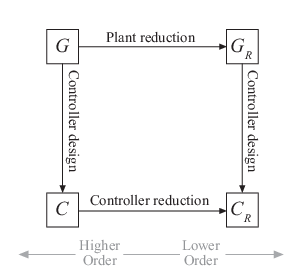Documentation

## Model Reduction Basics

Working with lower-order models can simplify analysis and control design, relative to higher-order models. Simpler models are also easier to understand and manipulate. High-order models obtained by linearizing complex Simulink® models or from other sources can contain states that do not contribute much to the dynamics of particular interest to your application. Therefore, it can be useful to reduce model order while preserving model characteristics that are important for your application.

### When to Reduce Model Order

Cases where you might want to reduce model order include these situations:

• You are working with a relatively high-order model obtained from linearizing a Simulink model, performing a finite-element calculation, interconnecting model elements, or other source.

• You want to improve the simulation speed of a Simulink model at a certain operating point. In that case, you can linearize a portion of the model at that operating point and compute a reduced-order simplification or approximation of the linearized model. You can then replace the portion of the model with an LTI Block containing the reduced-order model.

• You design a high-order controller that you want to implement as a lower-order controller, such as a PID controller. For example, controller design using Linear-Quadratic-Gaussian methods or H synthesis techniques can yield a high-order result. In this case, you can try reducing the plant order before synthesis, reducing the controller order after synthesis, or both.

• You want to simplify a model obtained by identification with System Identification Toolbox™ software.

The following diagram illustrates the relationship between model reduction and control design.In general, when designing a controller for a system represented by a high-order model, G, it is useful to start by simplifying the plant model. Then, design a relatively low-order controller, CR, for the lower-order plant model GR. After you design a controller for either the original or the reduced plant model, you can try to reduce the controller further.

Reducing the plant or controller can include:

• Discarding states that do not contribute to the system dynamics, such as structurally disconnected states or canceling pole-zero pairs.

• Discarding low-energy states that contribute relatively little to system dynamics.

• Focusing on a particular frequency region and discarding dynamics outside that region. For example, if your control bandwidth is limited by actuator dynamics, discard higher-frequency dynamics.

In any case, when you reduce model order, you want to preserve model characteristics that are important for your application. Whenever you compute a reduced-order model, verify that the reduced model preserves time-domain or frequency-domain behavior that you care about. For example, for control design, it is useful to verify that the reduced closed-loop system is stable. It is also useful to check that the reduced open-loop transfer function CRGR adequately matches the original models where the open-loop gain GC is close to 1 (in the gain crossover region).

### Model Reduction Tools

Control System Toolbox™ offers tools for model reduction in several environments. These include:

• Functions for performing model reduction at the MATLAB® command prompt, in scripts, or in your own functions.

• Reduce Model Order task for generating code in the Live Editor. When you are working in a live script, use this task to interactively experiment with model-reduction methods and parameters and generate code for your live script.

• Model Reducer app, a standalone app that lets you import models from the MATLAB workspace, and interactively generate reduced-order models using different methods and parameters. The app can also generate code for use in a MATLAB script or function.

### Choosing a Model Reduction Method

To reduce the order of a model, you can either simplify your model, or compute a lower-order approximation. The following table summarizes the differences among several model-reduction approaches.

ApproachCommand LineModel Reducer App and Reduce Model Order Live Editor Task
Simplification — Reduce model order exactly by canceling pole-zero pairs or eliminating states that have no effect on the overall model response
• `sminreal` — Eliminate states that are structurally disconnected from the inputs or outputs.

• `minreal` — Eliminate canceling or near-canceling pole-zero pairs from transfer functions. Eliminate unobservable or uncontrollable states from state-space models.

Pole-Zero Simplification method — Eliminate:

• Structurally disconnected states

• Unobservable or uncontrollable states from state-space models

• Canceling or near-canceling pole-zero pairs from transfer functions

Approximation — Compute a lower-order approximation of your model. `balred` — Discard states that have relatively low effect on the overall model response.Balanced Truncation method — Discard states that have relatively low effect on the overall model response.
Mode Selection — Eliminate poles and zeros that fall outside a specific frequency range of interest.`freqsep` — Separate model into slow and fast dynamics around a specified cutoff frequency.Mode Selection method — Select frequency range of interest and discard dynamics outside that range.

Sometimes, approximation can yield better results, even if the model looks like a good candidate for simplification. For example, models with near pole-zero cancellations are sometimes better reduced by approximation than simplification. Similarly, using `balred` to reduce state-space models can yield more accurate results than `minreal`.

When you use a reduced-order model, always verify that the simplification or approximation preserves model characteristics that are important for your application. For example, compare the frequency responses of the original and reduced models using `bodeplot` or `sigmaplot`. Or, compare the open-loop responses for the original and reduced plant and controller models.